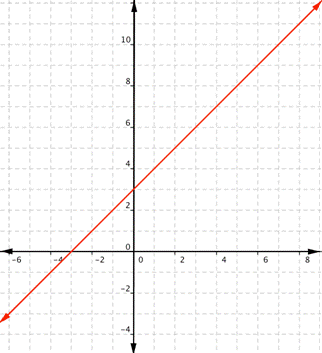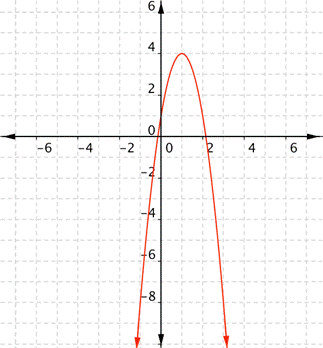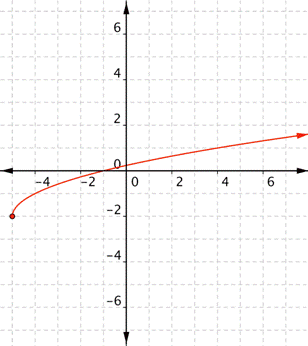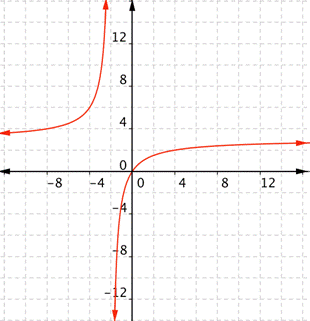## Finding Domain and Range From a Graph

### Learning Outcome

• Find the domain and range of a function from its graph

We have learned that the set of input values for a function is called the domain of the function and the set of output values is called the range of the function.  We have also learned how to algebraically determine the domain of a function.  It is important to be aware that the domain and range of a function are not necessarily the same. For example, the function $f\left(x\right)=-\dfrac{1}{\sqrt{x}}$ has the set of all positive real numbers as its domain but the set of all negative real numbers as its range. As a more extreme example, a function’s inputs and outputs can be completely different categories (for example, names of weekdays as inputs and numbers as outputs, as on an attendance chart), in such cases the domain and range have no elements in common.

Finding domain and range of different functions is often a matter of asking yourself, what values can this function not have? Pictures make it easier to visualize what the domain and range are, so we will show how to define the domain and range of functions given their graphs.

What are the domain and range of the real-valued function $f(x)=x+3$?
This is a linear function. Remember that linear functions are lines that continue forever in each direction.Any real number can be substituted for x and get a meaningful output. For any real number, you can always find an x value that gives you that number for the output. Unless a linear function is a constant, such as $f(x)=2$, there is no restriction on the range.
The domain and range are all real numbers.

For the examples that follow, try to figure out the domain and range of the graphs before you look at the answer.

### Example

What are the domain and range of the real-valued function $f(x)=−3x^{2}+6x+1$?### Example

What is the domain and range of the real-valued function $f(x)=-2+\sqrt{x+5}$?### Example

What is the domain of the real-valued function $\displaystyle f(x)=\frac{3x}{x+2}$?In the following video we show how to define the domain and range of functions from their graphs.

## Summary

Although a function may be given as “real valued,” it may be that the function has restrictions to its domain and range. There may be some real numbers that cannot be part of the domain or part of the range. This is particularly true with rational and radical functions which can have restrictions to their domain, range, or both. Other functions, such as quadratic functions and polynomial functions of even degree, can also have restrictions to their range.

## Contribute!

Did you have an idea for improving this content? We’d love your input.